Request a Free Counselling Session from our Expert Mentor# NCERT Solution for Class 12 Physics Chapter 13

Welcome to the NCERT Solutions for Class 12 Physics Chapter 13, provided by Swastik Classes. This chapter covers the topic of Nuclei, which deals with the study of the properties and behavior of atomic nuclei.

You will learn about the discovery of the atomic nucleus, its structure, and its composition. The chapter also covers the concept of nuclear forces and their properties. Additionally, you will learn about the different types of radioactive decay, including alpha, beta, and gamma decay.

Moreover, the chapter discusses the various applications of nuclear energy, including nuclear fission and fusion. You will learn about the chain reaction and critical mass, as well as the different types of nuclear reactors. The chapter also covers the different methods used for the disposal of nuclear waste.

Our NCERT Solutions for Class 12 Physics Chapter 13 provide step-by-step explanations and solutions to all the questions in the textbook. With our solutions, you can easily understand the concepts covered in the chapter and develop a deeper understanding of the subject.

## Answers of Physics NCERT solutions for class 12 Chapter 13 – Nuclei

Question 13.1:

(a) Two stable isotopes of lithium  and  have respective abundances of 7.5% and 92.5%. These isotopes have masses 6.01512 u and 7.01600 u, respectively. Find the atomic mass of lithium.

(b) Boron has two stable isotopes,  and Their respective masses are 10.01294 u and 11.00931 u, and the atomic mass of boron is 10.811 u. Find the abundances of   and

(a)  Mass of lithium isotope , m1 = 6.01512 u

Mass of lithium isotope , m2 = 7.01600 u

Abundance of , η1= 7.5%

Abundance of , η2= 92.5%

The atomic mass of lithium atom is given as:

(b)  Mass of boron isotope  m1 = 10.01294 u

Mass of boron isotope  m2 = 11.00931 u

Abundance of  η1 = x%

Abundance of  η2= (100 − x)%

Atomic mass of boron, m = 10.811 u

The atomic mass of boron atom is given as:

And 100 − x = 80.11%

Hence, the abundance of  is 19.89% and that of  is 80.11%.

*Question 13.2:

The three stable isotopes of neon:  and have respective abundances of 90.51%, 0.27% and 9.22%. The atomic masses of the three isotopes are 19.99 u, 20.99 u and 21.99 u, respectively. Obtain the average atomic mass of neon.

Atomic mass of , m1= 19.99 u

Abundance of , η= 90.51%

Atomic mass of , m= 20.99 u

Abundance of , η= 0.27%

Atomic mass of , m= 21.99 u

Abundance of , η3 = 9.22%

The average atomic mass of neon is given as:

Question 13.3:

Obtain the binding energy (in MeV) of a nitrogen nucleus given =14.00307 u

Atomic mass of nitrogen , m = 14.00307 u

A nucleus of nitrogen  contains 7 protons and 7 neutrons.

Hence, the mass defect of this nucleus, Δ= 7mH + 7mn − m

Where,

Mass of a proton, mH = 1.007825 u

Mass of a neutron, mn= 1.008665 u

∴Δm = 7 × 1.007825 + 7 × 1.008665 − 14.00307

= 7.054775 + 7.06055 − 14.00307

= 0.11236 u

But 1 u = 931.5 MeV/c2

∴Δ= 0.11236 × 931.5 MeV/c2

Hence, the binding energy of the nucleus is given as:

Eb = Δmc2

Where,

c = Speed of light

Eb = 0.11236 × 931.5

= 104.66334 MeV

Hence, the binding energy of a nitrogen nucleus is 104.66334 MeV.

*Question 13.4:

Obtain the binding energy of the nuclei  and  in units of MeV from the following data:

= 55.934939 u = 208.980388 u

Atomic mass of , m1 = 55.934939 u

nucleus has 26 protons and (56 − 26) = 30 neutrons

Hence, the mass defect of the nucleus, Δ= 26 × mH + 30 × mn − m1

Where,

Mass of a proton, mH = 1.007825 u

Mass of a neutron, mn = 1.008665 u

∴Δ= 26 × 1.007825 + 30 × 1.008665 − 55.934939

= 26.20345 + 30.25995 − 55.934939

= 0.528461 u

But 1 u = 931.5 MeV/c2

∴Δ= 0.528461 × 931.5 MeV/c2

The binding energy of this nucleus is given as:

Eb1 = Δmc2

Where,

c = Speed of light

Eb1 = 0.528461 × 931.5

= 492.26 MeV

Average binding energy per nucleon

Atomic mass of  m2 = 208.980388 u

nucleus has 83 protons and (209 − 83) 126 neutrons.

Hence, the mass defect of this nucleus is given as:

Δm‘ = 83 × mH + 126 × mn − m2

Where,

Mass of a proton, mH = 1.007825 u

Mass of a neutron, mn = 1.008665 u

∴Δm‘ = 83 × 1.007825 + 126 × 1.008665 − 208.980388

= 83.649475 + 127.091790 − 208.980388

= 1.760877 u

But 1 u = 931.5 MeV/c2

∴Δm‘ = 1.760877 × 931.5 MeV/c2

Hence, the binding energy of this nucleus is given as:

Eb2 = Δmc2

= 1.760877 × 931.5

= 1640.26 MeV

Average binding energy per nucleon =

Question 13.5:

A given coin has a mass of 3.0 g. Calculate the nuclear energy that would be required to separate all the neutrons and protons from each other. For simplicity assume that the coin is entirely made of  atoms (of mass 62.92960 u).

Mass of a copper coin, m’ = 3 g

Atomic mass of  atom, m = 62.92960 u

The total number of  atoms in the coin,

Where,

NA = Avogadro’s number = 6.023 × 1023 atoms /g

Mass number = 63 g

nucleus has 29 protons and (63 − 29) 34 neutrons

∴Mass defect of this nucleus, Δm‘ = 29 × mH + 34 × mn − m

Where,

Mass of a proton, mH = 1.007825 u

Mass of a neutron, mn = 1.008665 u

∴Δm‘ = 29 × 1.007825 + 34 × 1.008665 − 62.9296

= 0.591935 u

Mass defect of all the atoms present in the coin, Δm = 0.591935 × 2.868 × 1022

= 1.69766958 × 1022 u

But 1 u = 931.5 MeV/c2

∴Δ= 1.69766958 × 1022 × 931.5 MeV/c2

Hence, the binding energy of the nuclei of the coin is given as:

Eb = Δmc2

= 1.69766958 × 1022 × 931.5

= 1.581 × 1025 MeV

But 1 MeV = 1.6 × 10−13 J

Eb = 1.581 × 1025 × 1.6 × 10−13

= 2.5296 × 1012 J

This much energy is required to separate all the neutrons and protons from the given coin.

*Question 13.6:

Write nuclear reaction equations for

(i)  α-decay of  (ii)  α-decay of

(iii)  β−-decay of   (iv)  β-decay of

(v)  β+-decay of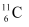(vi)  β+-decay of

(vii)  Electron capture of

α is a nucleus of helium and β is an electron (e− for β− and e+ for β+). In every α-decay, there is a loss of 2 protons and 4 neutrons. In every β+-decay, there is a loss of 1 proton and a neutrino is emitted from the nucleus. In every β−-decay, there is a gain of 1 proton and an antineutrino is emitted from the nucleus.

For the given cases, the various nuclear reactions can be written as:

Question 13.7:

A radioactive isotope has a half-life of years. How long will it take the activity to reduce to a) 3.125%, b) 1% of its original value?

Half-life of the radioactive isotope = T years

Original amount of the radioactive isotope = N0

(a)  After decay, the amount of the radioactive isotope = N

It is given that only 3.125% of Nremains after decay. Hence, we can write:

Where,

λ = Decay constant

t = Time

Hence, the isotope will take about 5T years to reduce to 3.125% of its original value.

(b)  After decay, the amount of the radioactive isotope = N

It is given that only 1% of Nremains after decay. Hence, we can write:

Since, λ = 0.693/T

Hence, the isotope will take about 6.645T years to reduce to 1% of its original value.

*Question 13.8:

The normal activity of living carbon-containing matter is found to be about 15 decays per minute for every gram of carbon. This activity arises from the small proportion of radioactive  present with the stable carbon isotope When the organism is dead, its interaction with the atmosphere (which maintains the above equilibrium activity) ceases and its activity begins to drop. From the known half-life (5730 years) of and the measured activity, the age of the specimen can be approximately estimated. This is the principle of  dating used in archaeology. Suppose a specimen from Mohenjodaro gives an activity of 9 decays per minute per gram of carbon. Estimate the approximate age of the Indus-Valley civilisation.

Decay rate of living carbon-containing matter, R = 15 decay/min

Let N be the number of radioactive atoms present in a normal carbon- containing matter.

Half life of  = 5730 years

The decay rate of the specimen obtained from the Mohenjodaro site:

R‘ = 9 decays/min

Let N’ be the number of radioactive atoms present in the specimen during the Mohenjodaro period.

Therefore, we can relate the decay constant, λand time, t as:

Hence, the approximate age of the Indus-Valley civilisation is 4223.5 years.

Question 13.9:

Obtain the amount of  necessary to provide a radioactive source of 8.0 mCi strength. The half-life of  is 5.3 years.

The strength of the radioactive source is given as:

Where,

N = Required number of atoms

Half-life of  = 5.3 years

= 5.3 × 365 × 24 × 60 × 60

= 1.67 × 108 s

For decay constant λ, we have the rate of decay as:

Where,

For :

Mass of 6.023 × 1023 (Avogadro’s number) atoms = 60 g

∴Mass of  atoms

Hence, the amount of  necessary for the purpose is 7.106 × 10−6 g.

Question 13.10:

The half-life of  is 28 years. What is the disintegration rate of 15 mg of this isotope?

Half life of  , = 28 years

= 28 × 365 × 24 × 60 × 60

= 8.83 × 108 s

Mass of the isotope, m = 15 mg

90 g of atom contains 6.023 × 1023 (Avogadro’s number) atoms.

Therefore, 15 mg of  contains:

Rate of disintegration,

Where,

λ = Decay constant

Hence, the disintegration rate of 15 mg of the given isotope is 7.878 × 1010 atoms/s.

*Question 13.11:

Obtain approximately the ratio of the nuclear radii of the gold isotope  and the silver isotope

Nuclear radius of the gold isotope  = RAu

Nuclear radius of the silver isotope  = RAg

Mass number of gold, AAu = 197

Mass number of silver, AAg = 107

The ratio of the radii of the two nuclei is related with their mass numbers as:

Hence, the ratio of the nuclear radii of the gold and silver isotopes is about 1.23.

Question 13.12:

Find the Q-value and the kinetic energy of the emitted α-particle in the α-decay of (a)  and (b)

Given

= 226.02540 u,  = 222.01750 u,

= 220.01137 u, = 216.00189 u.

(a)  Alpha particle decay of  emits a helium nucleus. As a result, its mass number reduces to (226 − 4) 222 and its atomic number reduces to (88 − 2) 86. This is shown in the following nuclear reaction.

Q-value of emitted α-particle = (Sum of initial mass − Sum of final mass) c2

Where,

c = Speed of light

It is given that:

Q-value = [226.02540 − (222.01750 + 4.002603)] u c2

= 0.005297 u c2

But 1 u = 931.5 MeV/c2

Q = 0.005297 × 931.5 ≈ 4.94 MeV

Kinetic energy of the α-particle

(b)  Alpha particle decay of  is shown by the following nuclear reaction.

It is given that:

Mass of = 220.01137 u

Mass of = 216.00189 u

Q-value =

≈ 6.41 MeV

Kinetic energy of the α-particle

= 6.29 MeV

Question 13.13:

The radionuclide 11C decays according to

The maximum energy of the emitted positron is 0.960 MeV.

Given the mass values:

calculate and compare it with the maximum energy of the positron emitted

The given nuclear reaction is:

Atomic mass of = 11.011434 u

Atomic mass of

Maximum energy possessed by the emitted positron = 0.960 MeV

The change in the Q-value (ΔQ) of the nuclear masses of the  nucleus is given as:

Where,

me = Mass of an electron or positron = 0.000548 u

= Speed of light

m’ = Respective nuclear masses

If atomic masses are used instead of nuclear masses, then we have to add 6 me in the case of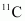and 5 me in the case of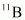.

Hence, equation (1) reduces to:

∴ΔQ = [11.011434 − 11.009305 − 2 × 0.000548] c2

= (0.001033 c2) u

But 1 u = 931.5 Mev/c2

∴ΔQ = 0.001033 × 931.5 ≈ 0.962 MeV

The value of Q is almost comparable to the maximum energy of the emitted positron.

*Question 13.14:

The nucleus  decays by emission. Write down the  decay equation and determine the maximum kinetic energy of the electrons emitted. Given that: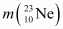= 22.994466 u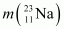= 22.989770 u.

In  emission, the number of protons increases by 1, and one electron and an antineutrino are emitted from the parent nucleus.

emission of the nucleus  is given as:

It is given that:

Atomic mass of  = 22.994466 u

Atomic mass of = 22.989770 u

Mass of an electron, me = 0.000548 u

Q-value of the given reaction is given as:

There are 10 electrons in  and 11 electrons in . Hence, the mass of the electron is cancelled in the Q-value equation.

The daughter nucleus is too heavy as compared to  and  Hence, it carries negligible energy. The kinetic energy of the antineutrino is nearly zero. Hence, the maximum kinetic energy of the emitted electrons is almost equal to the Q-value, i.e., 4.374 MeV.

Question 13.15:

The value of a nuclear reaction → is defined by

= [ mAmb− mC− md]cwhere the masses refer to the respective nuclei. Determine from the given data the Q-value of the following reactions and state whether the reactions are exothermic or endothermic.

(i)

(ii)

Atomic masses are given to be

(i)  The given nuclear reaction is:

It is given that:

Atomic mass

Atomic mass

Atomic mass

According to the question, the Q-value of the reaction can be written as:

The negativeQ-value of the reaction shows that the reaction is endothermic.

(ii)  The given nuclear reaction is:

It is given that:

Atomic mass of

Atomic mass of

Atomic mass of

The Q-value of this reaction is given as:

The positive Q-value of the reaction shows that the reaction is exothermic.

Question 13.16:

Suppose, we think of fission of a  nucleus into two equal fragments, Is the fission energetically possible? Argue by working out of the process. Given   and .

The fission of  can be given as:

It is given that:

Atomic mass of  = 55.93494 u

Atomic mass of

The Q-value of this nuclear reaction is given as:

The Q-value of the fission is negative. Therefore, the fission is not possible energetically. For an energetically-possible fission reaction, the Q-value must be positive.

Question 13.17:

The fission properties of  are very similar to those of .The average energy released per fission is 180 MeV. How much energy, in MeV, is released if all the atoms in 1 kg of pure  undergo fission?

Average energy released per fission of

Amount of pure , m = 1 kg = 1000 g

NA= Avogadro number = 6.023 × 1023

Mass number of = 239 g

1 mole of contains NA atoms.

m g of  contains

∴Total energy released during the fission of 1 kg of is calculated as:

Hence,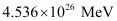is released if all the atoms in 1 kg of pure  undergo fission.

*Question 13.18:

A 1000 MW fission reactor consumes half of its fuel in 5.00 y. How much

did it contain initially? Assume that the reactor operates 80% of the time, that all the energy generated arises from the fission of  and that this nuclide is consumed only by the fission process.

Half life of the fuel of the fission reactor,  years

= 5 × 365 × 24 × 60 × 60 s

We know that in the fission of 1 g of nucleus, the energy released is equal to 200 MeV.

1 mole, i.e., 235 g of contains 6.023 × 1023 atoms.

∴1 g  contains atoms

The total energy generated per gram of is calculated as:

The reactor operates only 80% of the time.

Hence, the amount of consumed in 5 years by the 1000 MW fission reactor is calculated as:

∴Initial amount of = 2 × 1538 = 3076 kg

Question 13.19:

How long can an electric lamp of 100W be kept glowing by fusion of 2.0 kg of deuterium? Take the fusion reaction as

The given fusion reaction is:

Amount of deuterium, m = 2 kg

1 mole, i.e., 2 g of deuterium contains 6.023 × 1023 atoms.

∴2.0 kg of deuterium contains

It can be inferred from the given reaction that when two atoms of deuterium fuse, 3.27 MeV energy is released.

∴Total energy per nucleus released in the fusion reaction:

Power of the electric lamp, P = 100 W = 100 J/s

Hence, the energy consumed by the lamp per second = 100 J

The total time for which the electric lamp will glow is calculated as:

*Question 13.20:

Calculate the height of the potential barrier for a head on collision of two deuterons. (Hint: The height of the potential barrier is given by the Coulomb repulsion between the two deuterons when they just touch each other. Assume that they can be taken as hard spheres of radius 2.0 fm.)

When two deuterons collide head-on, the distance between their centres, d is given as:

Radius of a deuteron nucleus = 2 fm = 2 × 10−15 m

d = 2 × 10−15 + 2 × 10−15 = 4 × 10−15 m

Charge on a deuteron nucleus = Charge on an electron = e = 1.6 × 10−19 C

Potential energy of the two-deuteron system:

Where,

= Permittivity of free space

Hence, the height of the potential barrier of the two-deuteron system is 360 keV.

Question 13.21:

From the relation R0A1/3, where R0 is a constant and is the mass number of a nucleus, show that the nuclear matter density is nearly constant (i.e. independent of A).

We have the expression for nuclear radius as:

R0A1/3

Where,

R0 = Constant.

A = Mass number of the nucleus

Nuclear matter density,

Let m be the average mass of the nucleus.

Hence, mass of the nucleus = mA

Hence, the nuclear matter density is independent of A. It is nearly constant.

*Question 13.22:

For the  (positron) emission from a nucleus, there is another competing process known as electron capture (electron from an inner orbit, say, the K−shell, is captured by the nucleus and a neutrino is emitted).

Show that if  emission is energetically allowed, electron capture is necessarily allowed but not vice−versa.

Let the amount of energy released during the electron capture process be Q1. The nuclear reaction can be written as:

Let the amount of energy released during the positron capture process be Q2. The nuclear reaction can be written as:

= Nuclear mass of

= Nuclear mass of

= Atomic mass of

= Atomic mass of

me = Mass of an electron

= Speed of light

Q-value of the electron capture reaction is given as:

Q-value of the positron capture reaction is given as:

It can be inferred that if Q2 > 0, then Q> 0; Also, if Q1> 0, it does not necessarily mean that Q2 > 0.

In other words, this means that ifemission is energetically allowed, then the electron capture process is necessarily allowed, but not vice-versa. This is because the Q-value must be positive for an energetically-allowed nuclear reaction.

Question 13.23:

In a periodic table the average atomic mass of magnesium is given as 24.312 u. The average value is based on their relative natural abundance on earth. The three isotopes and their masses are  (23.98504u),

(24.98584u) and  (25.98259u). The natural abundance of is 78.99% by mass. Calculate the abundances of other two isotopes.

Average atomic mass of magnesium, m = 24.312 u

Mass of magnesium isotope , m1 = 23.98504 u

Mass of magnesium isotope , m= 24.98584 u

Mass of magnesium isotope, m= 25.98259 u

Abundance of , η1= 78.99%

Abundance of , ηx%

Hence, abundance of , η= 100 − x − 78.99% = (21.01 − x)%

We have the relation for the average atomic mass as:

Hence, the abundance of is 9.3% and that of is 11.71%.

*Question 13.24:

The neutron separation energy is defined as the energy required to remove a neutron from the nucleus. Obtain the neutron separation energies of the nuclei  and

from the following data:

= 39.962591 u

= 40.962278 u

= 25.986895 u

= 26.981541 u

For

For

A neutron  is removed from a  nucleus. The corresponding nuclear reaction can be written as:

It is given that:

Mass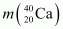= 39.962591 u

Mass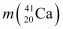) = 40.962278 u

Mass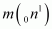= 1.008665 u

The mass defect of this reaction is given as:

Δm =

∴Δm = 0.008978 × 931.5 MeV/c2

Hence, the energy required for neutron removal is calculated as:

For , the neutron removal reaction can be written as:

It is given that:

Mass  = 26.981541 u

Mass = 25.986895 u

The mass defect of this reaction is given as:

Hence, the energy required for neutron removal is calculated as:

*Question 13.25:

A source contains two phosphorous radio nuclides  and  (T1/2 = 25.3d). Initially, 10% of the decays come from . How long one must wait until 90% do so?

Half life of , T1/2 = 14.3 days

Half life of , T’1/2 = 25.3 days

nucleus decay is 10% of the total amount of decay.

The source has initially 10% of nucleus and 90% of  nucleus.

Suppose after t days, the source has 10% of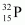nucleus and 90% of  nucleus.

Initially:

Number of nucleus = N

Number of nucleus = 9 N

Finally:

Number of nucleus = 9 N’

Number of  nucleus = N’

For  nucleus, we can write the number ratio as:

For , we can write the number ratio as:

On dividing equation (1) by equation (2), we get:

Hence, it will take about 208.5 days for 90% decay of

Question 13.26:

Under certain circumstances, a nucleus can decay by emitting a particle more massive than an α-particle. Consider the following decay processes:

Calculate the Q-values for these decays and determine that both are energetically allowed.

Take a  emission nuclear reaction:

We know that:

Mass of  m1 = 223.01850 u

Mass of  m2 = 208.98107 u

Mass of  m3 = 14.00324 u

Hence, the Q-value of the reaction is given as:

Q = (m1 − m2 − m3c2

= (223.01850 − 208.98107 − 14.00324) c2

= (0.03419 c2) u

But 1 u = 931.5 MeV/c2

Q = 0.03419 × 931.5

= 31.848 MeV

Hence, the Q-value of the nuclear reaction is 31.848 MeV. Since the value is positive, the reaction is energetically allowed.

Now take a  emission nuclear reaction:

We know that:

Mass of  m1 = 223.01850

Mass of m2 = 219.00948

Mass of , m3 = 4.00260

Q-value of this nuclear reaction is given as:

Q = (m1 − m2 − m3c2

= (223.01850 − 219.00948 − 4.00260) C2

= (0.00642 c2) u

= 0.00642 × 931.5 = 5.98 MeV

Hence, the Q value of the second nuclear reaction is 5.98 MeV. Since the value is positive, the reaction is energetically allowed.

Question 13.27:

Consider the fission of  by fast neutrons. In one fission event, no neutrons are emitted and the final end products, after the beta decay of the primary fragments, are  and . Calculate Q for this fission process. The relevant atomic and particle masses are

m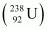=238.05079 u

m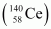=139.90543 u

m= 98.90594 u

In the fission of 10 β− particles decay from the parent nucleus. The nuclear reaction can be written as:

It is given that:

Mass of a nucleus m1 = 238.05079 u

Mass of a nucleus m2 = 139.90543 u

Mass of a nucleus  m3 = 98.90594 u

Mass of a neutron m4 = 1.008665 u

Q-value of the above equation,

Where,

m’ = Represents the corresponding atomic masses of the nuclei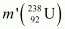m1 − 92me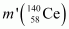m2 − 58me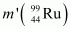m3 − 44me

m4

Hence, the Q-value of the fission process is 231.007 MeV.

*Question 13.28:

Consider the D−T reaction (deuterium−tritium fusion)

(a)  Calculate the energy released in MeV in this reaction from the data:

= 2.014102 u

= 3.016049 u

(b) Consider the radius of both deuterium and tritium to be approximately 2.0 fm. What is the kinetic energy needed to overcome the coulomb repulsion between the two nuclei? To what temperature must the gas be heated to initiate the reaction? (Hint: Kinetic energy required for one fusion event =average thermal kinetic energy available with the interacting particles = 2(3kT/2); = Boltzman’s constant, = absolute temperature.)

(a)  Take the D-T nuclear reaction:

It is given that:

Mass of , m1= 2.014102 u

Mass of , m= 3.016049 u

Mass of  m= 4.002603 u

Mass of  m= 1.008665 u

Q-value of the given D-T reaction is:

Q = [mm2− m3 − m4c2

= [2.014102 + 3.016049 − 4.002603 − 1.008665] c2

= [0.018883 c2] u

But 1 u = 931.5 MeV/c2

Q = 0.018883 × 931.5 = 17.59 MeV

(b)  Radius of deuterium and tritium, r ≈ 2.0 fm = 2 × 10−15 m

Distance between the two nuclei at the moment when they touch each other, d = r + r = 4 × 10−15 m

Charge on the deuterium nucleus = e

Charge on the tritium nucleus = e

Hence, the repulsive potential energy between the two nuclei is given as:

Where,

0 = Permittivity of free space

Hence, 5.76 × 10−14 J or of kinetic energy (KE) is needed to overcome the Coulomb repulsion between the two nuclei.

However, it is given that:

Where,

k = Boltzmann constant = 1.38 × 10−23 m2 kg s−2 K−1

T = Temperature required for triggering the reaction

Hence, the gas must be heated to a temperature of 1.39 × 109 K to initiate the reaction.

*Question 13.29:

Obtain the maximum kinetic energy of β-particles, and the radiation frequencies of γ decays in the decay scheme shown in Fig. 13.6. You are given that

(198Au) = 197.968233 u

(198Hg) =197.966760 u

It can be observed from the given γ-decay diagram that γ1 decays from the 1.088 MeV energy level to the 0 MeV energy level.

Hence, the energy corresponding to γ1-decay is given as:

E1 = 1.088 − 0 = 1.088 MeV

1= 1.088 × 1.6 × 10−19 × 106 J

Where,

h = Planck’s constant = 6.6 × 10−34 Js

It can be observed from the given γ-decay diagram that γ2 decays from the 0.412 MeV energy level to the 0 MeV energy level.

Hence, the energy corresponding to γ2-decay is given as:

E2 = 0.412 − 0 = 0.412 MeV

2= 0.412 × 1.6 × 10−19 × 106 J

Where,

It can be observed from the given γ-decay diagram that γ3 decays from the 1.088 MeV energy level to the 0.412 MeV energy level.

Hence, the energy corresponding to γ3-decay is given as:

E3 = 1.088 − 0.412 = 0.676 MeV

3= 0.676 × 10−19 × 106 J

Where,

Mass of = 197.968233 u

Mass of = 197.966760 u

1 u = 931.5 MeV/c2

Energy of the highest level is given as:

βdecays from the 1.3720995 MeV level to the 1.088 MeV level

∴Maximum kinetic energy of the βparticle = 1.3720995 − 1.088

= 0.2840995 MeV

βdecays from the 1.3720995 MeV level to the 0.412 MeV level

∴Maximum kinetic energy of the βparticle = 1.3720995 − 0.412

= 0.9600995 MeV

Question 13.30:

Calculate and compare the energy released by a) fusion of 1.0 kg of hydrogen deep within Sun and b) the fission of 1.0 kg of 235U in a fission reactor.

(a)  Amount of hydrogen, m = 1 kg = 1000 g

1 mole, i.e., 1 g of hydrogen contains 6.023 × 1023 atoms.

∴1000 g of  contains 6.023 × 1023 × 1000 atoms.

Within the sun, four  nuclei combine and form one  nucleus. In this process 26 MeV of energy is released.

Hence, the energy released from the fusion of 1 kg  is:

(b)  Amount of  = 1 kg = 1000 g

1 mole, i.e., 235 g of  contains 6.023 × 1023 atoms.

∴1000 g of contains  atoms

It is known that the amount of energy released in the fission of one atom of is 200 MeV.

Hence, energy released from the fission of 1 kg of  is:

Therefore, the energy released in the fusion of 1 kg of hydrogen is nearly 8 times the energy released in the fission of 1 kg of uranium.

*Question 13.31:

Suppose India had a target of producing by 2020 AD, 200,000 MW of electric power, ten percent of which was to be obtained from nuclear power plants. Suppose we are given that, on an average, the efficiency of utilization (i.e. conversion to electric energy) of thermal energy produced in a reactor was 25%. How much amount of fissionable uranium would our country need per year by 2020? Take the heat energy per fission of 235U to be about 200MeV.

Amount of electric power to be generated, P = 2 × 105 MW

10% of this amount has to be obtained from nuclear power plants.

∴Amount of nuclear power,

= 2 × 104 MW

= 2 × 104 × 106 J/s

= 2 × 1010 × 60 × 60 × 24 × 365 J/y

Heat energy released per fission of a 235U nucleus, E = 200 MeV

Efficiency of a reactor = 25%

Hence, the amount of energy converted into the electrical energy per fission is calculated as:

Number of atoms required for fission per year:

1 mole, i.e., 235 g of U235 contains 6.023 × 1023 atoms.

∴Mass of 6.023 × 1023 atoms of U235 = 235 g = 235 × 10−3 kg

∴Mass of 78840 × 1024 atoms of U235

Hence, the mass of uranium needed per year is 3.076 × 104 kg.

## Physics Class 12 Chapter 13 NCERT Solutions – Nuclei

NCERT Class 12 Physics Chapter 13 deals with the close study of an atom, specifically the nucleus. It goes on to explain in detail the nuclear composition, binding forces, and other facts and studies about the atom/nucleus. The unit also covers radioactivity as a nuclear phenomenon. Energy is required for sustaining life and we can’t depend on conventional sources for too long. It is for this reason that non-conventional sources like nuclear energy, wind energy, etc. are being extensively used nowadays. The chapter also covers nuclear reactions used in the processes of nuclear fission and fusion in atomic reactors to produce energy for mass use. Chapter 12 and 13 of the NCERT cover the study of atoms, their forces, and their properties in depth.

## FAQs on Class 12 Physics Chapter 13

### What are nuclei?

Nuclei (plural for nucleus). The nucleus is the central part of the atom that consists of protons and neutrons.

### What are nuclear fission and fusion?

Nuclear reactions such as fission and fusion both produce energy, but the processes are extremely different. Fission is the process of a heavy, unstable nucleus splitting into two lighter nuclei, while fusion is the process of two light nuclei combining to release massive amounts of energy.

## Summary

1. The density of nuclear matter is unaffected by the nucleus’ size. The atom’s mass density does not follow this rule.
1. The radius of a nucleus measured by electron scattering differs slightly from the radius measured by alpha-particle scattering. This is since electron scattering detects the nucleus’ charge distribution, whereas alpha and related particles detect nuclear matter.
1. After Einstein demonstrated the equivalence of mass and energy, E = mc2, we can no longer speak of distinct laws of mass and energy conservation; instead, we must speak of a single law of mass and energy conservation. Nuclear physics provides the most compelling evidence that this principle operates in nature. It’s crucial to our understanding of nuclear energy and how we can use it to generate electricity. Q of a nuclear process (decay or reaction) can also be represented in terms of beginning and final masses using this method.
1. When two light nuclei fuse or a heavy nucleus undergoes fission into nuclei of intermediate-mass, the nature of the binding energy (per nucleon) curve indicates that exothermic nuclear processes are feasible.
1. The light nuclei must have enough initial energy to overcome the coulomb potential barrier to fuse. As a result, fusion necessitates extremely high temperatures.

Conclusion

The NCERT Solutions for Class 12 Physics Chapter 13 provided by Swastik Classes cover all the important concepts related to Nuclei. The solutions provide step-by-step explanations of all the questions given in the textbook, making it easier for students to understand the concepts and solve the problems.

The chapter covers the discovery of the atomic nucleus, its structure, and its composition. It also covers the concept of nuclear forces and their properties. Additionally, the solutions discuss the different types of radioactive decay, including alpha, beta, and gamma decay, along with their characteristics.

Moreover, the chapter covers the different applications of nuclear energy, including nuclear fission and fusion. You will learn about the chain reaction and critical mass, as well as the different types of nuclear reactors. The chapter also covers the various methods used for the disposal of nuclear waste.

Our solutions are designed to help students develop a better understanding of the subject and prepare them for their exams. The step-by-step explanations and solutions provided in the solutions make it easier for students to learn the concepts and solve the problems with ease.

Overall, the NCERT Solutions for Class 12 Physics Chapter 13 are an excellent resource for students who want to develop a strong foundation in the subject of Nuclei. With these solutions, students can improve their problem-solving skills and achieve academic success.